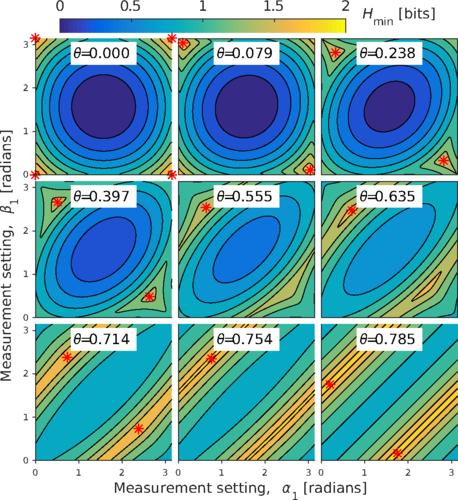# Quantum random number generation

The laws of quantum mechanics do not dictate a definite outcome for a measurement—only the probabilities of each outcome. This makes it ideal source of random numbers. Additionally, continuous variable (CV) systems have a high bandwidth and efficient detectors compared with their discrete variable counterpart. The ANU quantum optics group have been conducting a series of experiments to demonstrate this.

#### Trusted quantum random number generators

In 2011, Thomas Symul demonstrated a real time high bit rate quantum random number (QRN) generation with a coherent laser light . We called this the listening to the sounds of silence. With a bit rate of 2 Gbps, this the world’s fastest real time demonstration at the time. In 2012, the random numbers from this setup was made available online. The randomness are converted to sounds and graphics that you can listen and see. Every random number on the website are generated from the quantum properties of the vacuum and served only once.

In 2015, Jing Yan Haw performed a theoretical and experimental study at how to maximise the amount of extractable randomness for a CV-QRN generator . This was achieved by optimising the how the continuous measurement are discretised into binary ones and zeros. The amount of secure randomness is determined by the conditional min-entropy of the measured sequence. A detailed explanation on the CV-QRN generator was published in this book chapter .

#### Random numbers from a Bell measurementIn 2016, Syed Assad studied how to maximise the device-independent randomness originating from a discrete variable Bell experiment . Given a certain experimentally prepared state, the optimal measurements that will give the highest amount of secure randomness was found through an iterative algorithm.

#### Machine learning algorithm for attacking a random number generator

In 2019, Duy Truong Nhan develop a predictive machine learning analysis to investigate the impact of deterministic classical noise in different stages of an optical CV-QRN generator . This demonstrates that appropriate filtering and randomness extraction processes are necessary in order for the generated numbers to be truly random.

#### Source device independent random number generatorIn 2019, Thibault Michel built a real-time source independent QRN generator . Unlike a trusted source QRN generator, a source independent QNR generator does not require the user to trust the quantum source. Instead, the measurement basis is continuously switched to quantify the amount of randomness available in the source. In this experiment, the SI-QRN generator was tested using both a squeezed source and a thermal source.

#### References

 T. Symul, S. Assad, and P. Lam, “Real time demonstration of high bitrate quantum random number generation with coherent laser light,” Appl. Phys. Lett., vol. 98, no. 23, pp. 231103–231103, 2011.

 J. Y. Haw et al., “Maximization of extractable randomness in a quantum random-number generator,” Physical Review Applied, vol. 3, no. 5, May 2015.

 J. Y. Haw, S. M. Assad, and P. K. Lam, “Secure Random Number Generation in Continuous Variable Systems,” In: Kollmitzer C., Schauer S., Rass S., Rainer B. (eds) Quantum Random Number Generation, Quantum Science and Technology, 2020.

 S. M. Assad, O. Thearle, and P. K. Lam, “Maximizing device-independent randomness from a bell experiment by optimizing the measurement settings,” Physical Review A, vol. 94, no. 1, p. 012304, 2016.

 N. D. Truong, J. Y. Haw, S. M. Assad, P. K. Lam, and O. Kavehei, “Machine learning cryptanalysis of a quantum random number generator,” IEEE Transactions on Information Forensics and Security, vol. 14, no. 2, pp. 403–414, 2018.

 T. Michel et al., “Real-time source independent quantum random number generator with squeezed states,” Physical Review Applied 12 (3), 034017 2019.